# Give parametric equations that describe a full circle of radius​ R, centered at the origin with...

Give parametric equations that describe a full circle of radius​ R, centered at the origin with clockwise​ orientation, where the parameter t varies over the interval ​[0,22​]. Assume that the circle starts at the point​ (R,0) along the​ x-axis.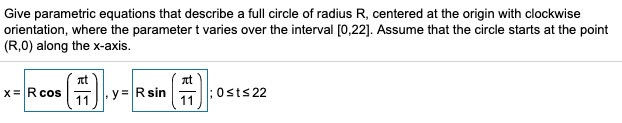Consider the following parametric​ equations, x=−t+7​, y=−3t−3​; minus−5less than or equals≤tless than or equals≤5. Complete parts​ (a) through​ (d) below.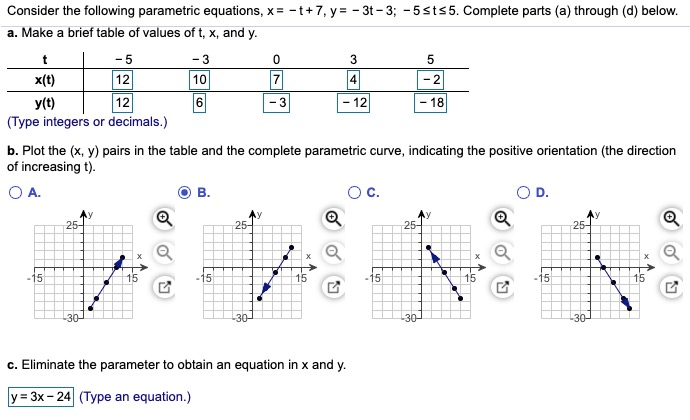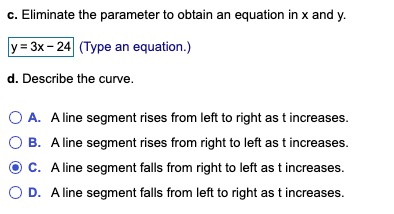Consider the following parametric equation.

a.Eliminate the parameter to obtain an equation in x and y.

b.Describe the curve and indicate the positive orientation.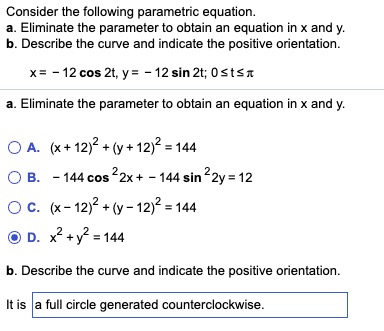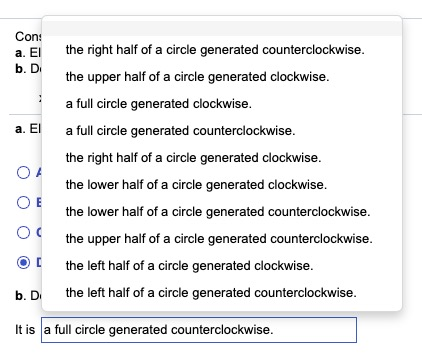Consider the following parametric equations.

a. Eliminate the parameter to obtain an equation in x and y.

b. Describe the curve and indicate the positive orientation.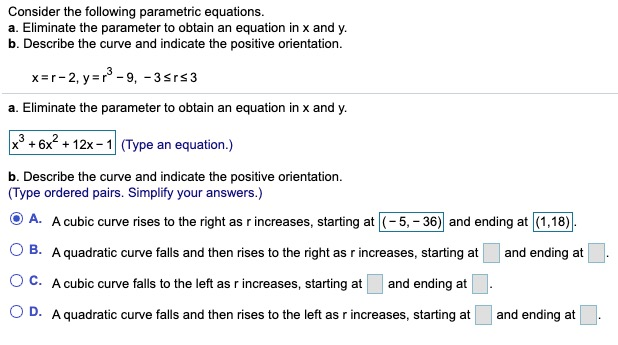Eliminate the parameter to express the following parametric equations as a single equation in x and y. x=tant​, y=sec^2t-1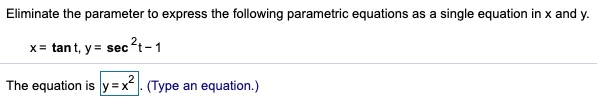Give parametric equations that describe a full circle of radius R, centered at the origin with clockwise orientation, where the parametert varies over the interval [0,22]. Assume that the circle starts at the point (R.0) along the x-axis. x= 10 cos () Rsin (.):osts22
Consider the following parametric equations, x = -t+7, y = - 3t-3; -5 sts5. Complete parts (a) through (d) below. a. Make a brief table of values of t, x, and y. tl -5 0 X(t) 12 10 7 y(t) | |12 -3 - 12 - 18 (Type integers or decimals.) 4 b. Plot the (x, y) pairs in the table and the complete parametric curve, indicating the positive orientation (the direction of increasing t). OA. ch on 21 30 30 -30 c. Eliminate the parameter to obtain an equation in x and y. y = 3x – 24 (Type an equation.)
c. Eliminate the parameter to obtain an equation in x and y. y = 3x - 24 (Type an equation.) d. Describe the curve. OA. A line segment rises from left to right as t increases. O B. A line segment rises from right to left as t increases. OC. A line segment falls from right to left as t increases. O D. A line segment falls from left to right as t increases.
Consider the following parametric equation. a. Eliminate the parameter to obtain an equation in x and y. b. Describe the curve and indicate the positive orientation. x= -12 cos 2t, y= -12 sin 2t; O sts a. Eliminate the parameter to obtain an equation in x and y. O A. (x + 12)2 + (y + 12)2 = 144 OB. - 144 cos 22x + = 144 sin 22y = 12 O C. (x – 12)2 + (y - 12)2 = 144 D. x² + y2 = 144 b. Describe the curve and indicate the positive orientation. It is a full circle generated counterclockwise.
Con: a. El bD the right half of a circle generated counterclockwise. the upper half of a circle generated clockwise a full circle generated clockwise. a full circle generated counterclockwise. the right half of a circle generated clockwise. the lower half of a circle generated clockwise. the lower half of a circle generated counterclockwise. the upper half of a circle generated counterclockwise. the left half of a circle generated clockwise. the left half of a circle generated counterclockwise b. D. It is a full circle generated counterclockwise.
Consider the following parametric equations. a. Eliminate the parameter to obtain an equation in x and y. b. Describe the curve and indicate the positive orientation. x=r-2, y=p2-9, -35153 a. Eliminate the parameter to obtain an equation in x and y. x3 + 6x2 + 12x-1 (Type an equation.) b. Describe the curve and indicate the positive orientation. (Type ordered pairs. Simplify your answers.) O A. A cubic curve rises to the right as r increases, starting at (-5, -36) and ending at (1,18) B. A quadratic curve falls and then rises to the right as r increases, starting at and ending at . O O C. A cubic curve falls to the left as r increases, starting at and ending at D. A quadratic curve falls and then rises to the left as r increases, starting at . and ending at O
Eliminate the parameter to express the following parametric equations as a single equation in x and y. x = tant, y = sec ?t-1 The equation is y=x?). (Type an equation.)

This Homework Help Question: "Give parametric equations that describe a full circle of radius​ R, centered at the origin with..." No answers yet.

We need 10 more requests to produce the answer to this homework help question. Share with your friends to get the answer faster!

0 /10 have requested the answer to this homework help question.

Once 10 people have made a request, the answer to this question will be available in 1-2 days.
All students who have requested the answer will be notified once they are available.

#### Earn Coin

Coins can be redeemed for fabulous gifts.

Similar Homework Help Questions
• ### Describe the shape and orientation (direction of positive motion) of the following parametric curve: X =...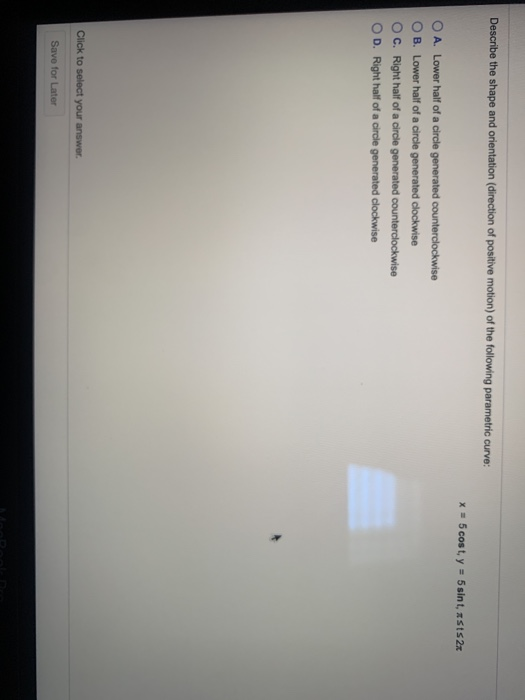Describe the shape and orientation (direction of positive motion) of the following parametric curve: X = 5 cost, y = 5 sint, SS2x O A. Lower half of a circle generated counterclockwise B. Lower half of a circle generated clockwise C. Right half of a circle generated counterclockwise OD. Right half of a circle generated clockwise Click to select your answer. Save for Later

• ### Consider the following parametric equations. x = V1 + 2 y = 2/t; Osts 16 a....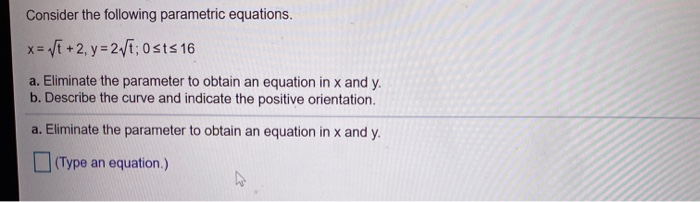Consider the following parametric equations. x = V1 + 2 y = 2/t; Osts 16 a. Eliminate the parameter to obtain an equation in x and y. b. Describe the curve and indicate the positive orientation. a. Eliminate the parameter to obtain an equation in x and y. (Type an equation)

• ### Please make it clear what to write in the box and which one is the right...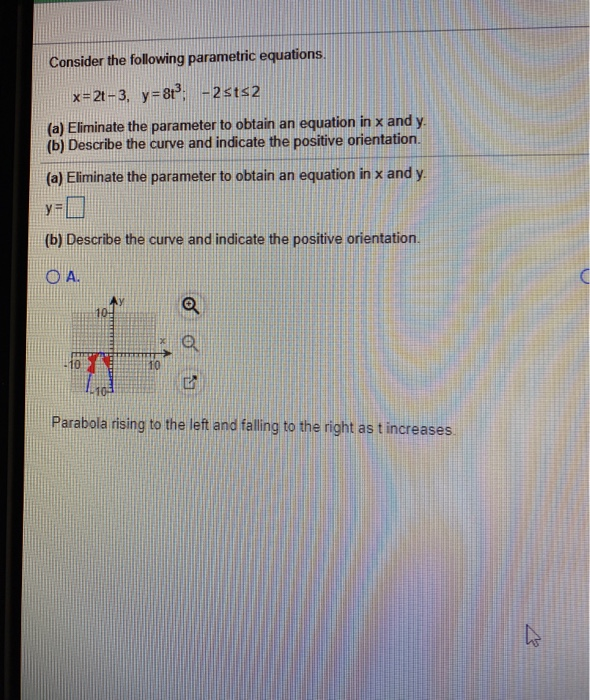Please make it clear what to write in the box and which one is the right answer by saying for example the answer is a Consider the following parametric equations. x=2t-3, y = 8 - 2sts2 (a) Eliminate the parameter to obtain an equation in x and y (b) Describe the curve and indicate the positive orientation. (a) Eliminate the parameter to obtain an equation in x and y. (b) Describe the curve and indicate the positive orientation. OA. Parabola...

• ### 12.1.23 Consider the following parametric equations. a. Eliminate the parameter to obtain an equation in x...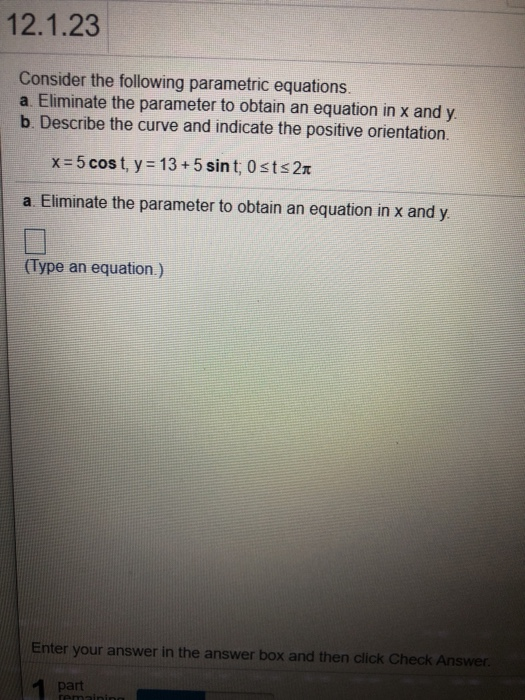12.1.23 Consider the following parametric equations. a. Eliminate the parameter to obtain an equation in x and y b. Describe the curve and indicate the positive orientation. x=5 cost, y = 13 + 5 sint; Osts 21 a. Eliminate the parameter to obtain an equation in x and y. (Type an equation.) Enter your answer in the answer box and then click Check Answer. part remini

• ### The position of an object in circular motion is modeled by the given parametric equations. Describe...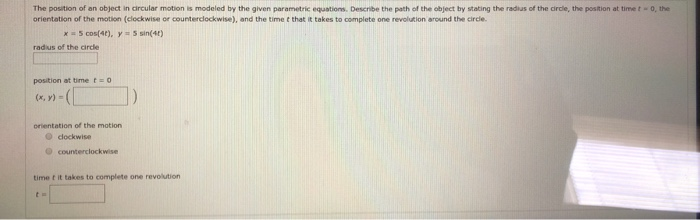The position of an object in circular motion is modeled by the given parametric equations. Describe the path of the object by stating the radius of the circle, the position at time to the orientation of the motion (clockwise or counterclockwise), and the time that it takes to complete one revolution around the circle. x = 5 cos(4), y = sin(40) radius of the circle position at time to (x, y) = orientation of the motion dockwise counterclockwise time it...

• ### Find parametric equations (not unique) for the following circle and give an interval for the parameter....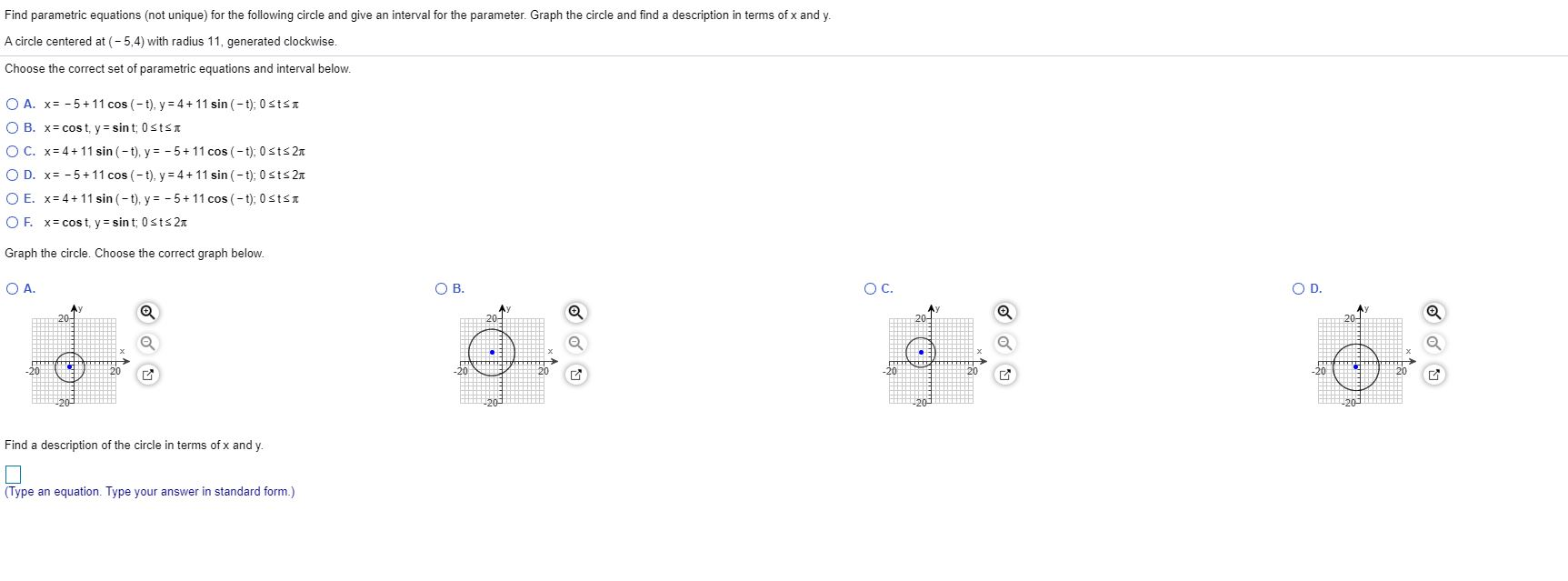Find parametric equations (not unique) for the following circle and give an interval for the parameter. Graph the circle and find a description in terms of x and y. A circle centered at (-5,4) with radius 11, generated clockwise. Choose the correct set of parametric equations and interval below. O A. x= -5+11 cos(-t), y = 4 + 11 sin(-t): 0 SISI OB. x= cost, y = sint: Ostst OC. x= 4 + 11 sin(-t), y = -5 + 11...

• ### Select the first set of parametric equations, x = a cos(bt), y = c sin(dt). (a)...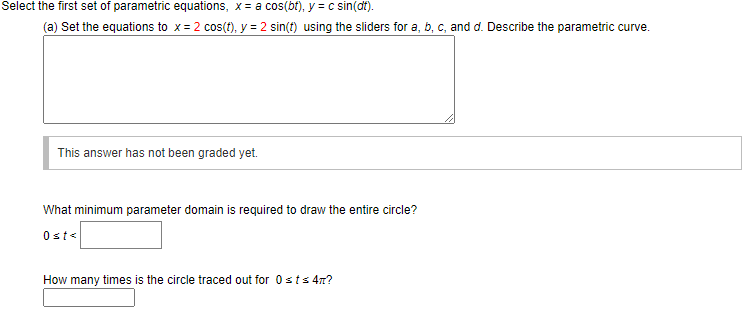Select the first set of parametric equations, x = a cos(bt), y = c sin(dt). (a) Set the equations to x = 2 cos(t), y = 2 sin(t) using the sliders for a, b, c, and d. Describe the parametric curve. This answer has not been graded yet. What minimum parameter domain is required to draw the entire circle? Osts How many times is the circle traced out for Osts 4? Click the Animate button and observe the relationship between...

• ### Consider the parametric equations below x-vi y-2.1 (a) Sketch the curve by using the parametric equations...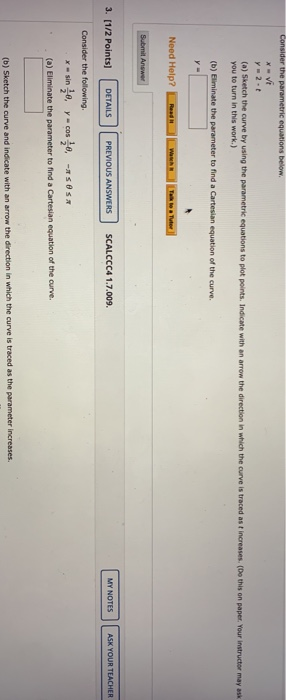Consider the parametric equations below x-vi y-2.1 (a) Sketch the curve by using the parametric equations to plot points. Indicate with an arrow the direction in which the curve is traced as t increases. (Do this on paper. Your instructor may ask you to turn in this work.) (b) Eliminate the parameter to find a Cartesian equation of the curve. y- Need Help? Submit Answer 3. [1/2 points) DETAILS PREVIOUS ANSWERS SCALCCC4 1.7.009. MY NOTES ASK YOUR TEACHER Consider the...

• ### Consider the parametric equations below. x = 2 + 4t y = 1-2 (a) Sketch the...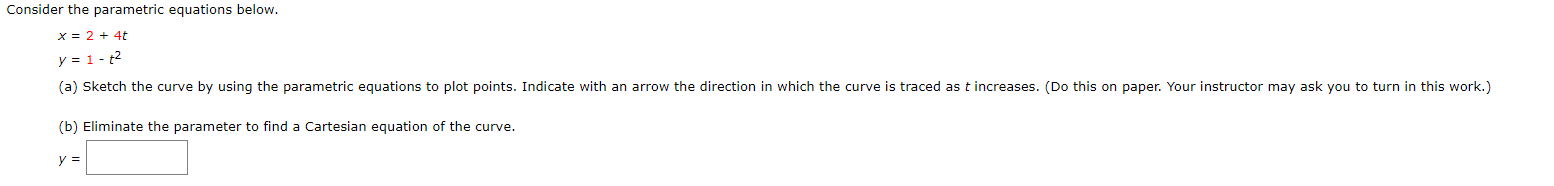Consider the parametric equations below. x = 2 + 4t y = 1-2 (a) Sketch the curve by using the parametric equations to plot points. Indicate with an arrow the direction in which the curve is traced as t increases. (Do this on paper. Your instructor may ask you to turn in this work.) (b) Eliminate the parameter to find a Cartesian equation of the curve. y = Consider the parametric equations below. x = 3t - 5 y =...

• ### Find the parametric equation of a circle of radius R, centered at (x = a; y...

Find the parametric equation of a circle of radius R, centered at (x = a; y = b), using the arc length as a parameter.